# Non Verbal Reasoning - Series

### Exercise :: Series - Section 1

Each of the following questions consists of five figures marked A, B, C, D and E called the Problem Figures followed by five other figures marked 1, 2, 3, 4 and 5 called the Answer Figures. Select a figure from amongst the Answer Figures which will continue the same series as established by the five Problem Figures.

46.

Select a figure from amongst the Answer Figures which will continue the same series as established by the five Problem Figures.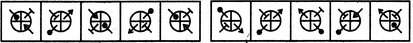(A)     (B)     (C)     (D)     (E)       (1)     (2)     (3)     (4)     (5)

 A. 1 B. 2 C. 3 D. 4 E. 5

Explanation:

All the elements move one space ACW (each space is equal to a quadrant of the circle) and get inverted in each step.

47.

Select a figure from amongst the Answer Figures which will continue the same series as established by the five Problem Figures.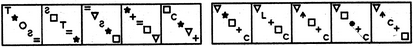(A)     (B)     (C)     (D)     (E)       (1)     (2)     (3)     (4)     (5)

 A. 1 B. 2 C. 3 D. 4 E. 5

Explanation:

In each step, the symbols move in the sequence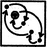and the symbol that reaches the encircled position gets replaced by a new one.

48.

Select a figure from amongst the Answer Figures which will continue the same series as established by the five Problem Figures.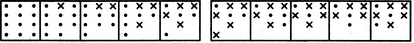(A)     (B)     (C)     (D)     (E)       (1)     (2)     (3)     (4)     (5)

 A. 1 B. 2 C. 3 D. 4 E. 5

Explanation:

In each step, one dot is lost while another dot is replaced by a cross.

49.

Select a figure from amongst the Answer Figures which will continue the same series as established by the five Problem Figures.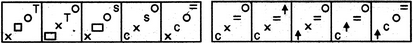(A)     (B)     (C)     (D)     (E)       (1)     (2)     (3)     (4)     (5)

 A. 1 B. 2 C. 3 D. 4 E. 5

Explanation:

The elements move in the sequences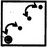and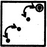alternately. Also, in each step, the element that reaches the encircled position, gets replaced by a new element.

50.

Select a figure from amongst the Answer Figures which will continue the same series as established by the five Problem Figures.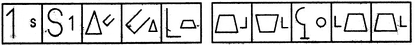(A)     (B)     (C)     (D)     (E)       (1)     (2)     (3)     (4)     (5)

 A. 1 B. 2 C. 3 D. 4 E. 5